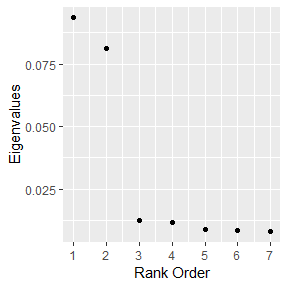# Introduction

In general, dann will struggle as unrelated variables are intermingled with related variables. To deal with this, sub_dann projects the data onto a unique subspace and then calls dann. The number of features in the subspace is controlled by the numDim argument. sub_dann is able to mitigate the use of noise variables. See section 3 of Discriminate Adaptive Nearest Neighbor Classification for details. Section 4 compares dann and sub_dann to a number of other approaches.

# Arguments

• k - The number of points in the neighborhood. Identical to k in standard k nearest neighbors. Same as dann function.
• neighborhood_size - The number of data points used to estimate class boundaries. Same as dann function.
• epsilon - Softening parameter. Usually has the least affect on performance. Same as dann function.
• weighted - Should the individual between class covariance matrices be weighted? FALSE corresponds to original publication.
• sphere - Type of covariance matrix to calculate.

# Example: Circle Data With Random Variables

In the below example there are 2 related variables and 5 that are unrelated. Lets see how dann, sub_dann, and dann with only the correct features perform.

 library(dann)
library(mlbench)
library(magrittr)
library(dplyr, warn.conflicts = FALSE)
library(ggplot2)

######################
# Circle data with unrelated variables
######################
set.seed(1)
train <- mlbench.circle(500, 2) %>%
tibble::as_tibble()
colnames(train)[1:3] <- c("X1", "X2", "Y")

# Add 5 unrelated variables
train <- train %>%
mutate(
U1 = runif(500, -1, 1),
U2 = runif(500, -1, 1),
U3 = runif(500, -1, 1),
U4 = runif(500, -1, 1),
U5 = runif(500, -1, 1)
)

xTrain <- train %>%
select(X1, X2, U1, U2, U3, U4, U5) %>%
as.matrix()

yTrain <- train %>%
pull(Y) %>%
as.numeric() %>%
as.vector()

test <- mlbench.circle(500, 2) %>%
tibble::as_tibble()
colnames(test)[1:3] <- c("X1", "X2", "Y")

# Add 5 unrelated variables
test <- test %>%
mutate(
U1 = runif(500, -1, 1),
U2 = runif(500, -1, 1),
U3 = runif(500, -1, 1),
U4 = runif(500, -1, 1),
U5 = runif(500, -1, 1)
)

xTest <- test %>%
select(X1, X2, U1, U2, U3, U4, U5) %>%
as.matrix()

yTest <- test %>%
pull(Y) %>%
as.numeric() %>%
as.vector()

dannPreds <- dann(xTrain, yTrain, xTest, 3, 50, 1, FALSE)
mean(dannPreds == yTest) # Not a good model
##  0.668

As expected, dann was not performant. Moving on to sub_dann, the dimension of the subspace should be chosen based on the number of large eigenvalues. The graph suggests 2 is good (the correct answer).

 graph_eigenvalues(xTrain, yTrain, 50, FALSE, "mcd")subDannPreds <- sub_dann(xTrain, yTrain, xTest, 3, 50,
1, FALSE, FALSE,
"mcd", 2)
mean(subDannPreds == yTest) # sub_dan does much better when unrelated variables are present.
##  0.882

sub_dann did much better than dann. Lets see how dann does if only related variables are used.

 variableSelectionDann <- dann(xTrain[, 1:2], yTrain, xTest[, 1:2], 3, 50, 1, FALSE)

mean(variableSelectionDann == yTest) # Best model found when only true predictors are used.
##  0.944

Overall, dann with the correct variables did better than sub_dann. In simulations one can simply pick the correct variables. In real applications the correct variables are usually unknown. sub_dann was able to estimate the correct number of features and get reasonably close to dann that only used related variables without having to know which variables are truly predictive.

 rm(train, test)
rm(xTrain, yTrain)
rm(xTest, yTest)
rm(dannPreds, subDannPreds, variableSelectionDann)